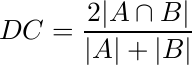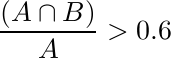This workshop is partially supported
by Australian Research Council,
discovery project DP140102794 &
ARC Future Fellowship (FT110100623).## Evaluation Metric

The quantitative performance of individual cell cytoplasm segmentation (on the synthetic dataset) is assessed using the average Dice Coefficient, measured asover the ‘‘good“ cell segmentations , where a ‘‘good” segmentation is considered to be one with a DC>0.7. We also measure the object-based false negative rate (FNo) obtained as the proportion of cells having a DC <= 0.7. In addition, we show a pixel-based evaluation using the true positive rate (TPp) and false positive rate (FPp) for both training and test sets using the ‘‘good" cell segmentations.

In addition, we assess the nuclei detection methodology using the criteria developed by Aksoy et al. . Specifically, first the precision and recall of nuclei detection is computed by considering the detection region A and (ground-truth) annotation B, and noting that a correct detection is represented by :andSecond, we compute the pixel-based precision and recall values of the correct detections using the Dice Coefficient .

The results file should be readable by the evaluation code, which considers an image as an member of a ‘‘cell’’ in Matlab. Each cell of the same image are the member of that image ‘‘cell’’. All the segmentation results of cells are in the form of binary masks.

References
1. Radau, P., Lu, Y., Connelly, K., Paul, G., Dick, A., Wright, G. Evaluation framework for algorithms segmenting short axis cardiac MRI. The MIDAS Journal - Cardiac MR Left Ventricle Segmentation Challenge (2009)

2. Gençtav, A., Aksoy, S., Önde, S. Unsupervised segmentation and classification of cervical cell images. Pattern Recognition, Vol. 45 (2012) 4151-4168.